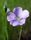## How to use a coumn from PROC SQL later as a variable?

Hello,

Using PROC SQL, I selected a column as, let's say, X. Now I want to use X as a variable later, in different procedures (e.g., PROC GLM). I tried to just use it but SAS doesn't recognize X as a variable. What should I do, so that SAS would recognize it as a variable?

1 ACCEPTED SOLUTION

Accepted Solutions

## Re: How to use a coumn from PROC SQL later as a variable?

PROC SQL without a CREATE TABLE statement prints a data set it does not create any data so the variables are not available for use.If you want the variable available later you need to create a table with the data.

``````proc sql;
create table stepXXXX as
select
(N_mean + 10)/50 as LN,
(1 - N1_mean)*10 as LN1
from OUTSTAT_N;
quit;
proc corr data= stepXXXX  plots = matrix(histogram);
var LN LN1;
run;``````
5 REPLIES 5

## Re: How to use a coumn from PROC SQL later as a variable?

There are too many possibilities for anybody to guess at the solution.  Show what you actually did, and it's likely that the solution is simple.

## Re: How to use a coumn from PROC SQL later as a variable?

Here is an example of what I am trying to do. In general, what I am asking about is how do I use columns in PROC SQL later in different procedures? I need to specify "data = " in later PROC statements (e.g., proc corr in this example). But it's not data in proc sql, it's just a table...

``````data MC (keep = N N1 sampleID);
call streaminit(12345678);
do sampleID = 1 to 1000;
do i = 1 to 10;
N1 = rand('Normal');
N2 = N1*5 + 15;
N = floor(N2);
output;
end;
end;
run;
proc means data = MC;
by sampleID;
var N N1;
output out = OUTSTAT_N mean = N_mean N1_mean;
run;
proc sql;
select
(N_mean + 10)/50 as LN,
(1 - N1_mean)*10 as LN1
from OUTSTAT_N;
quit;
proc corr data= ??? plots = matrix(histogram);
var LN LN1;
run;``````

## Re: How to use a coumn from PROC SQL later as a variable?

PROC SQL without a CREATE TABLE statement prints a data set it does not create any data so the variables are not available for use.If you want the variable available later you need to create a table with the data.

``````proc sql;
create table stepXXXX as
select
(N_mean + 10)/50 as LN,
(1 - N1_mean)*10 as LN1
from OUTSTAT_N;
quit;
proc corr data= stepXXXX  plots = matrix(histogram);
var LN LN1;
run;``````

## Re: How to use a coumn from PROC SQL later as a variable?

That was easy -- thank you!LinusH
Tourmaline | Level 20

## Re: How to use a coumn from PROC SQL later as a variable?

CREATE TABLE?
Data never sleeps
Discussion stats
• 5 replies
• 764 views
• 0 likes
• 4 in conversation